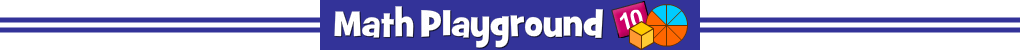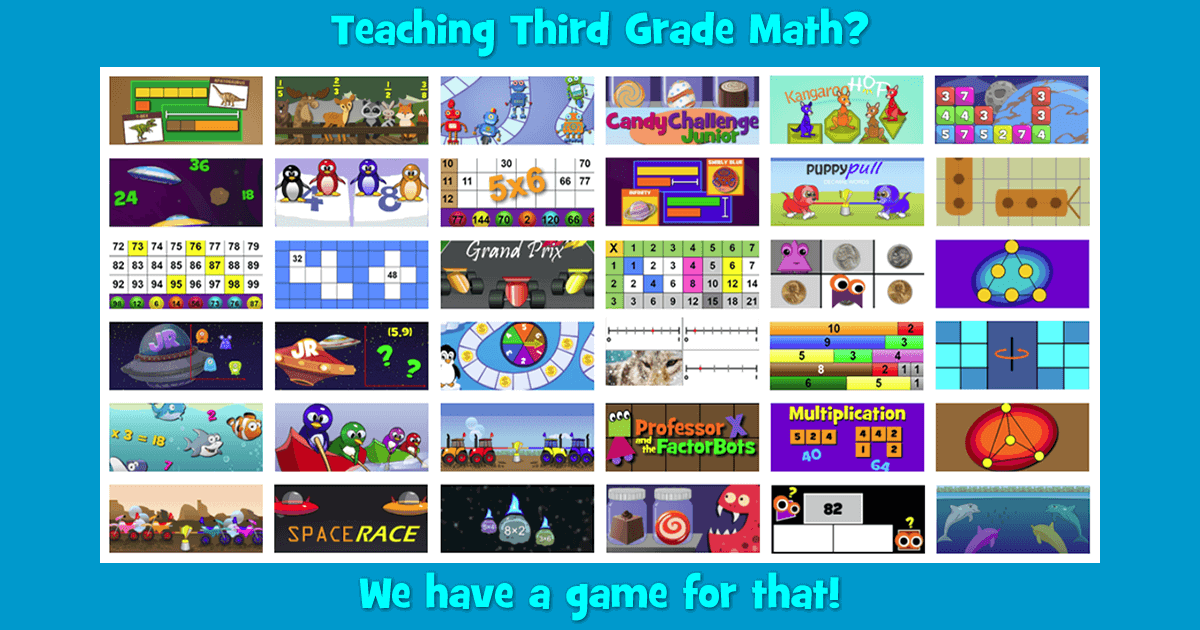Third grade marks a crucial year in the progression of students’ math skills. Students begin to visualize numbers in new ways. Equal groups and rectangular arrays are introduced. They venture beyond whole numbers to explore fractions and decimals. They begin to understand and quantify characteristics of shapes such as perimeter and area. Logical thinking, problem solving and organizational abilities often improve during 3rd grade. This makes it possible to include a broad range of mathematical tasks in your curriculum. The number of Aha! moments increases as your students begin to make connections between the math concepts they’re learning. In this blog, we’ll look at three major areas in the 3rd grade math curriculum and discuss ways to support your lesson plans.

##### Multiplication and Division
3rd grade students begin their investigation of multiplication by creating equal groups (for example, 3 groups of 5 objects) and relating that collection of objects to the expression 3 × 5. From there, students learn they can make a rectangular arrangement of those objects called an array. Properties of multiplication arise naturally from these representations. 3 groups of 5 objects can be organized into 5 groups of 3 objects. Students learn that 3 × 5 = 5 × 3 and that this relationship is the commutative property of multiplication. Arithmetic patterns can be explored through the use of a multiplication table. You students may discover that multiples of 5 always end in zero or five and multiples of 4 are always even. In a similar way, division is introduced and students begin to see the relationship between multiplication and division. By the end of 3rd grade math, students are expected to multiply and divide fluently within 100.

Math Playground has an extensive library of games to help students develop multiplication and division skills. Product Blocks is an excellent way for students to practice using the array model of multiplication. Students are given 3 factors and the corresponding arrays. The object of the game is to fill more of the grid with arrays than your opponent. You may project our interactive Multiplication Chart onto your whiteboard and ask students to identify patterns. Students may also explore factors, multiples, and square numbers. Help your students develop fact fluency with games such as Penguin Jump and Grand Prix Multiplication. Students can even compete with their classmates. Challenge your students to use multiplication and division to solve a myriad of interesting problems. Try algebraic reasoning in the Sweet Shop and escape the board game in Professor X and the Factorbots.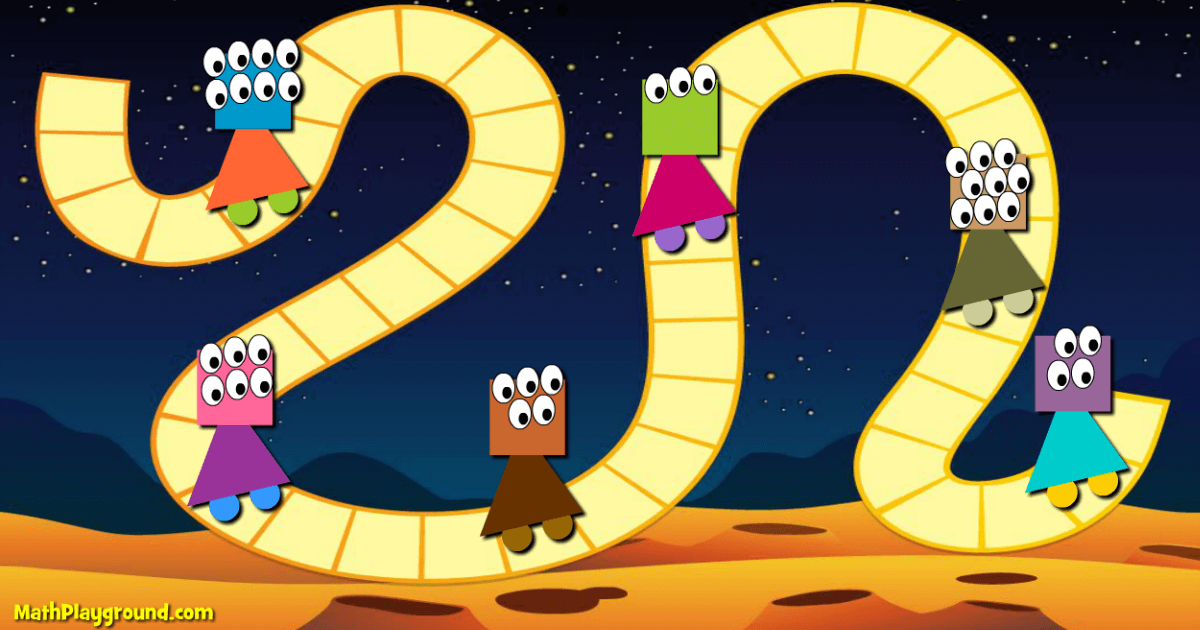Professor X and the Factorbots
##### Fractions
Students are introduced to unit fractions about halfway through 3rd grade math. They learn that a unit fraction is made when a whole is divided into some number of equal parts. From this unit fraction, other non-unit fractions can be composed. Students apply these ideas when exploring fractions on a number line. For example, students can locate the position of 3/7 by dividing the interval from 0 to 1 into seven equal parts and counting the first three consecutive parts. Third grade students are encouraged to reason about the relative sizes of fractions and identify fractions that are equivalent. Find ways to get your students talking about fractions. Listen to those conversations and identify any misconceptions.

Math Playground has developed an entire series of games around the concept of unit fractions. Fraction Forest guides students through the woods where they encounter forest animals struggling to understand fractions. Fargo and Denny introduces the idea of dividing a whole into equal parts. Later, students must copy a single part to make a larger non-unit fraction. In Part 2, students are challenged to decompose non-unit fractions and use those pieces to build new types of fractions. For example, students must consider how to transform a 2/9 fraction bar into a 7/10 fraction bar. It’s easy once students have learned the process. In the next set of Fraction Forest games, students explore equivalent fractions. In Part 1, they investigate unit fractions while Part 2 encourages students to explore non-unit fractions. In addition to the games, there are Unit Fraction and Equivalent Fraction playgrounds that you can use to introduce the challenges to your whole class.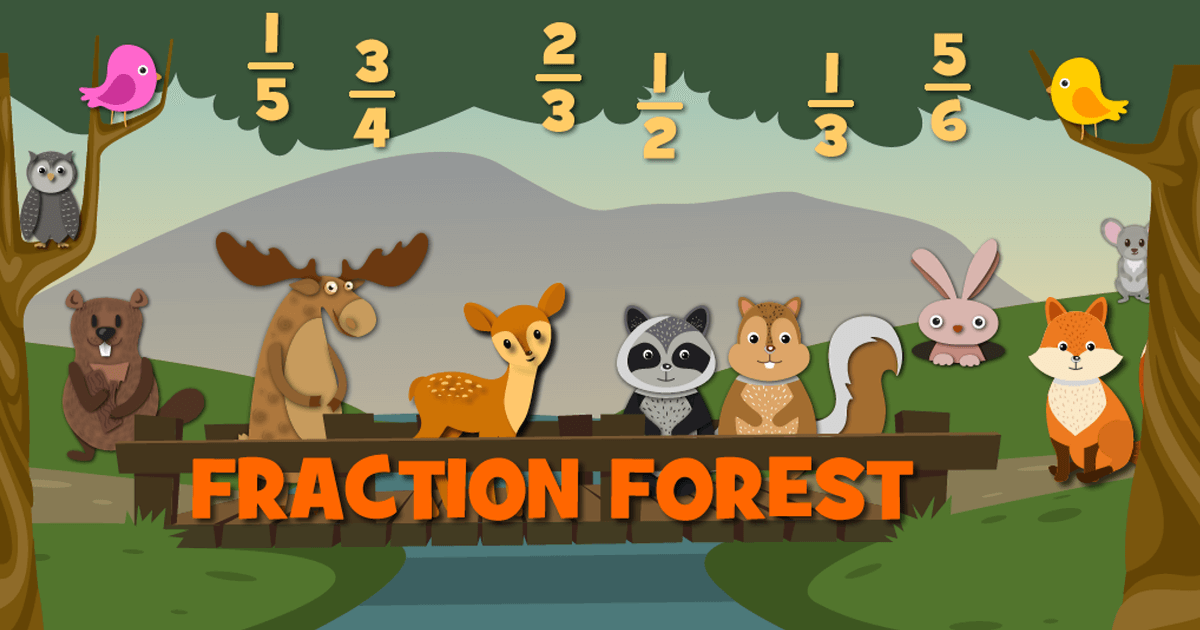the woodland animals of Fraction Forest
##### Area and Perimeter
Geometric measurement flows perfectly from the earlier work students have done in their multiplication unit, specifically their investigation of arrays. Students learn that the multiplication facts associated with arrays also describe the area of rectangles. Similarly, students can relate their fraction work to the geometry they are exploring. They find areas of complex figures by decomposing them into smaller parts. The area of one part is some fraction of the entire shape’s area.

Ask your students to build rectangular shapes with our interactive geoboard. You can project this tool onto your whiteboard and ask students how they would find the area. Students can also work independently. A writing tool is provided for students to keep track of measurements and calculations. Area Blocks challenges students to build simple and complex rectangular areas based on clues provided in the game. Our Pattern Blocks tool can be used to help students visualize fractional parts of shapes.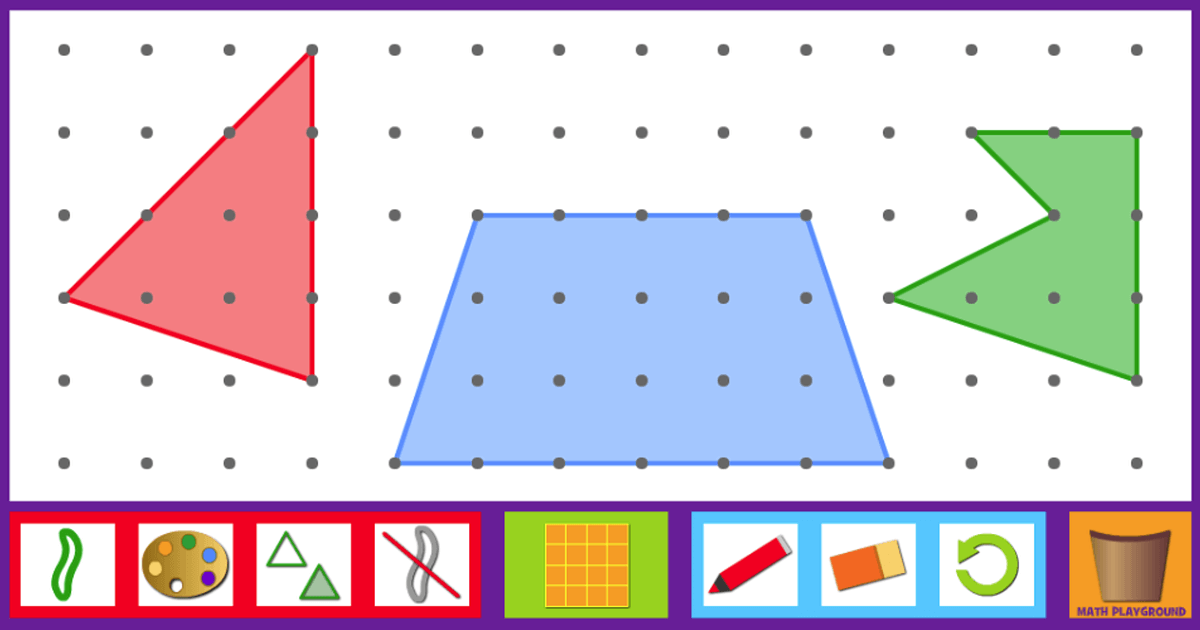interactive geometry board
Those of us who teach 3rd grade math are laying the foundation upon which all future math concepts rest. We can rise to the task by giving our students a variety of tools and activities that clarify ideas and provide new ways of visualizing mathematical relationships. We can provide challenges that encourage our students to apply basic concepts to more difficult tasks and encourage them to look beneath the surface. And we can accomplish all of these things in an engaging way with a plethora of games that are both educational and fun.

Try some math games in your classroom:
Product Blocks
Professor X and the Factorbots
Multiplication Chart
Penguin Jump
Grand Prix Multiplication
Sweet Shop
Fraction Forest
Fraction Forest Playground
Geometry Board
Area Blocks